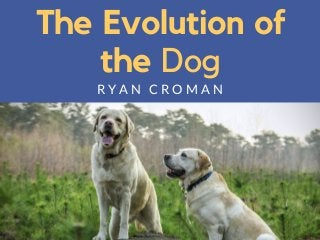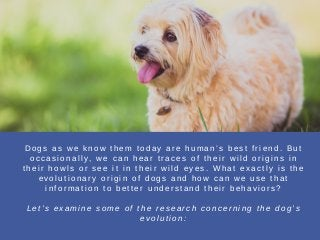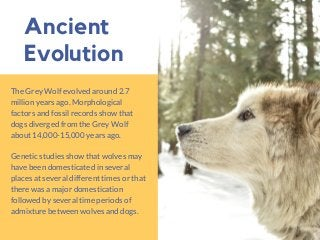Seu SlideShare está sendo baixado. ×

# The Evolution of the Dog | Ryan Croman

Anúncio
Anúncio
Anúncio
Anúncio
Anúncio
Anúncio
Anúncio
Anúncio
Anúncio
Anúncio
AnúncioCarregando em…3
×

1 de 8 Anúncio

# The Evolution of the Dog | Ryan Croman

Ryan Croman explains and discusses The Evolution of the Dog. For more from Ryan Croman, please visit his website: http://ryancroman.com

Ryan Croman explains and discusses The Evolution of the Dog. For more from Ryan Croman, please visit his website: http://ryancroman.com

Anúncio
Anúncio

### The Evolution of the Dog | Ryan Croman

1. 1. The Evolution of the Dog R Y A N C R O M A N
2. 2. D o g s a s w e k n o w t h e m t o d a y a r e h u m a n ’ s b e s t f r i e n d . B u t o c c a s i o n a l l y , w e c a n h e a r t r a c e s o f t h e i r w i l d o r i g i n s i n t h e i r h o w l s o r s e e i t i n t h e i r w i l d e y e s . W h a t e x a c t l y i s t h e e v o l u t i o n a r y o r i g i n o f d o g s a n d h o w c a n w e u s e t h a t i n f o r m a t i o n t o b e t t e r u n d e r s t a n d t h e i r b e h a v i o r s ? L e t ’ s e x a m i n e s o m e o f t h e r e s e a r c h c o n c e r n i n g t h e d o g ’ s e v o l u t i o n :
3. 3. Ancient Evolution The Grey Wolf evolved around 2.7 million years ago. Morphological factors and fossil records show that dogs diverged from the Grey Wolf about 14,000-15,000 years ago.  Genetic studies show that wolves may have been domesticated in several places at several different times or that there was a major domestication followed by several time periods of admixture between wolves and dogs.
4. 4. From Wolf to Dog   M a n y a t t r i b u t e s o f w o l v e s w e r e s p e c i f i c a l l y s e l e c t e d a n d b r e d t o e n h a n c e t r a i t s i n s p e c i f i c d o g b r e e d s . I n f a c t , a b e h a v i o r a l s t u d y f o u n d t h a t o f 9 0 d o g b e h a v i o r s , a l l b u t 1 9 t r a i t s w e r e o b s e r v e d i n w o l v e s . T h e m i s s i n g b e h a v i o r s w e r e o b s e r v e d a s m i n o r a c t i v i t i e s . S i m i l a r b o d y p o s t u r e s i n d i c a t i n g m o o d , l i k e a d o g l o w e r i n g i t s f r o n t b o d y t o i n d i c a t e p l a y f u l a t t i t u d e , s t e m s f r o m t h e i r o r i g i n s a s w o l v e s .
5. 5. Domestication Overtime, domestic dogs adopted tame behaviors that wild wolves do not display. Essentially, they are adult animals that retain playful behavior and dependence on humans and morphology, called neoteny. They maintained their smaller size, remain playful towards humans, and learned how to read human body language and expressions, building strong bonds with their dog owners.
6. 6. Dog Breeds Some dogs have retained strong wolf-like traits which have been bred for certain functions: hunting dogs, territorial guard dogs, scent dogs, and racing dogs. For the past 150 years, dogs have been bred for different reasons. Today, there are about 150 dog breeds in existence. Many people interbreed dogs to be pure bred.
7. 7. Eternal Bonds The evolution of the dog has transformed the mammal. Theories on dog’s evolution are continuously being rethought, and molecular testing methods are helping to determine evolutionary relationships and chronologies. Yet, one certainty is that the dog’s bond with humans is ancient and can withstand the sands of time.